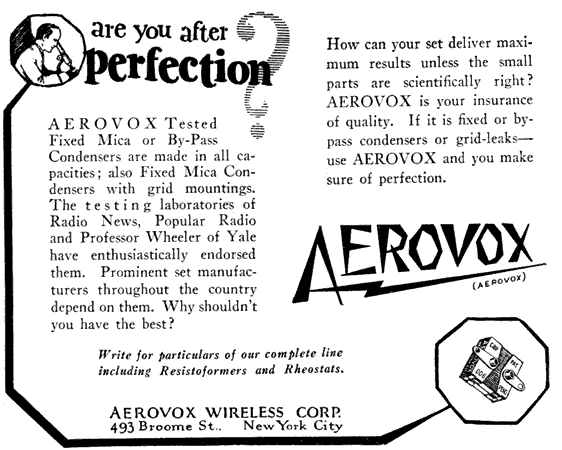## capacitor codes

Large capacitors have their values printed on them in plain terms, such as 100 uF. (We use the letter u as a substitute for the Greek letter micron, so "100 uF" represents 100 micro-farads.)

Small capacitors often use a 3-digit code to indicate their value. This code is similar to the resistor color code scheme, except that it uses digits instead of colored stripes. The first two characters are the 1st and 2nd significant digits, and the third digit is a multiplier. Values are expressed in pico-farads.

 third digit meaning 0 multiply by 1 1 multiply by 10 2 multiply by 100 3 multiply by 1,000 4 multiply by 10,000 5 multiply by 100,000 8 divide by 100 9 divide by 10

Examples:
 code meaning 221 220 pF 222 2200 pF 223 22,000 pF = .022 uF 224 220,000 pF = .22 uF 225 2,200,000 pF = 2.2 uF 228 0.22 pF 229 2.2 pF

If there are only 2 digits printed on a small disc capacitor, this is likely to be the value written directly in pico-farads, such as "47" = 47 pF. There is some ambiguity with 3-digit numbers ending in zero; "220" might mean 22 times 1, or it might mean 220.

Letters are used to indicate tolerances. For example, a cap marked 102J has a nominal value of 1000 pF and a +/- 5% tolerance. It can have an actual capacitance as low as 950 or as high as 1050 pF.

 letter tolerance F +/- 1% G +/- 2% H +/- 3% J +/- 5% K +/- 10% M +/- 20%

Capacitors sometimes have a letter-number-letter code, such as Z5U or X7R. This code indicates the minimum and maximum temperatures at which the cap is rated to function, and the amount of change in capacitance that will occur across that range of temperatures. As a general rule, you won't need this information unless you are planning to operate your equipment at near-freezing or near-boiling temperatures.

Capacitors marked NPO are designed to have minimal fluctuations of value over a normal range of operating temperatures. This high stability is desireable in oscillator circuits, but is generally not necessary in other capacitor applications.

Capacitors sometimes have a maximum voltage rating printed on them also. Generally speaking, tiny capacitors cannot endure high voltages, so if a cap is too small to have space for a voltage rating to be printed on it, assume that the maximum working voltage is 50 V or less.Hosted by www.Geocities.ws